Main Content

# RF Propagation and Visualization

This example shows how to compute and visualize outdoor wireless coverage between a transmitter and receiver. This includes:

• Transmitter and receiver site creation using Antenna Toolbox antenna library.

• Distance and angle calculation between the transmitter and the receiver.

• Signal strength computation using free space propagation or other propagation models.

• Communication link visualization.

• Transmitter coverage area calculation.

• Signal-to-interference plus noise ratio (SINR) calculation at the receiver.

Close `Site Viewer` if open before running the example.

### Transmitter Site

A transmitter consists of an RF circuit and an antenna, where the RF circuit excites the antenna with a signal and power. Key characteristics of a transmitter include its output power, operating frequency, and its antenna radiation pattern.

Use the `txsite` object to create a transmitter site at MathWorks Apple Hill campus. The latitude of the campus is 42.3001 degrees and the longitude is -71.3504 degrees.

```tx = txsite('Name','MathWorks Apple Hill',... 'Latitude',42.3001, ... 'Longitude',-71.3504)```
```tx = txsite with properties: Name: 'MathWorks Apple Hill' Latitude: 42.3001 Longitude: -71.3504 Antenna: 'isotropic' AntennaAngle: 0 AntennaHeight: 10 SystemLoss: 0 TransmitterFrequency: 1.9000e+09 TransmitterPower: 10 ```
`show(tx)`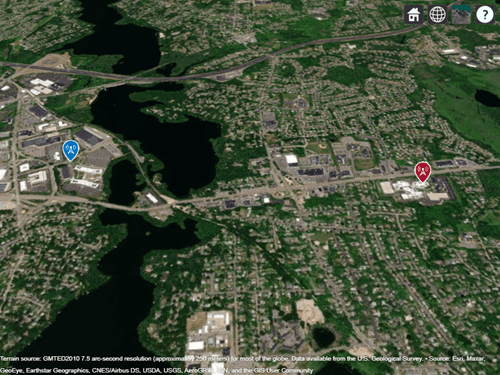### Receiver Site

A receiver consists of an RF circuit and an antenna, where the antenna intercepts radio waves and converts them to a current that is decoded by the RF circuit (e.g. demodulated) into a signal. Key characteristics of a receiver include its sensitivity and its antenna radiation pattern.

Use the `rxsite` object to create a receiver site at MathWorks Lakeside campus. The latitude of the campus is 42.3021 degrees and the longitude is -71.3764 degrees.

Receiver Sensitivity is the minimum signal power level that is necessary for a receiver to accurately decode a signal. Sensitivity is a power quantity and typically specified in dBm. To determine if a receiver will be in the coverage area of a transmitter, the sensitivity is compared to the received power.

```rx = rxsite('Name','MathWorks Lakeside', ... 'Latitude',42.3021, ... 'Longitude',-71.3764)```
```rx = rxsite with properties: Name: 'MathWorks Lakeside' Latitude: 42.3021 Longitude: -71.3764 Antenna: 'isotropic' AntennaAngle: 0 AntennaHeight: 1 SystemLoss: 0 ReceiverSensitivity: -100 ```
`show(rx)`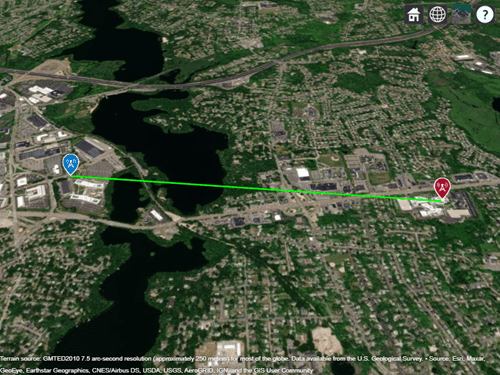### Distance Between Sites

Use the `distance` function to calculate the distance between the transmitter site and the receiver site. By default, this function draws a straight line between the transmitter and the receiver sites to calculate the distance. This path is called the Euclidean path and ignores all obstructions including the earth.

Another path option for the distance function is the great circle path, where the distance is calculated considering the curvature of the earth.

`dm = distance(tx,rx) % Unit: m`
```dm = 2.1556e+03 ```
`dkm = dm / 1000`
```dkm = 2.1556 ```

### Angle Between Sites

Use the `angle` function to calculate the angle between the transmitter site and the receiver site.

`azFromEast = angle(tx,rx) % Unit: degrees counter-clockwise from East`
```azFromEast = 174.0753 ```
`azFromNorth = -azFromEast + 90 % Convert angle to clockwise from North`
```azFromNorth = -84.0753 ```

### Received Power and Link Margin

The received power is given by the equation:

`${\mathit{P}}_{\mathrm{rx}}={\mathit{P}}_{\mathrm{tx}}+{\mathit{G}}_{\mathrm{tx}}+{\mathit{G}}_{\mathrm{rx}}-\mathrm{pathloss}$`

where,

• ${\mathit{P}}_{\mathrm{rx}}$ = Power available at the receiver.

• ${\mathit{P}}_{\mathrm{tx}}$ = Transmitter output power.

• ${\mathit{G}}_{\mathrm{tx}}$ = Transmitter gain.

• ${\mathit{G}}_{\mathrm{rx}}$ = Receiver gain.

• pathloss = RF attenuation suffered by the transmitter signal when it arrives at the receiver.

Signal strength is the signal level that is available at the receiver at a particular distance and angle from the transmitter. It is also known as received signal strength. Signal strength can be a power quantity or a voltage quantity.

Use the `sigstrength` function to calculate the signal strength of the transmitter at the receiver site. You can calculate two types of signal strength: Power and Electric field (e-field).

`ss = sigstrength(rx,tx)`
```ss = -67.0767 ```

Link margin is a measure of robustness of the communication link, calculated by subtracting the required receiver sensitivity from the signal strength available at the receiver.

`margin = abs(rx.ReceiverSensitivity - ss)`
```margin = 32.9233 ```

### Communication Link on Map

Use the `link `function to plot the success of the communication between the two sites on a map. The success of the link depends on the power received by the receiver from the transmitter.

By default, a green link shows you that power received meets or exceeds the receiver sensitivity. A red link shows unsuccessful communication between the transmitter and receiver sites.

`link(rx,tx)`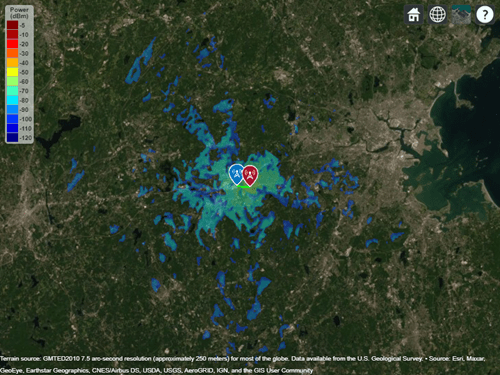### Coverage Map of Transmitter Site

A coverage map is the visualization of the geographic service area of the transmitter, which is where the received signal strength for a reference receiver meets its sensitivity. A coverage map may depict signal strength as either a power quantity (typically dBm) or a voltage quantity (typically dB$\mu$V/m).

Use the `coverage `function to calculate the coverage of the transmitter.

```coverage(tx,'close-in', ... 'SignalStrengths',-100:5:-60)```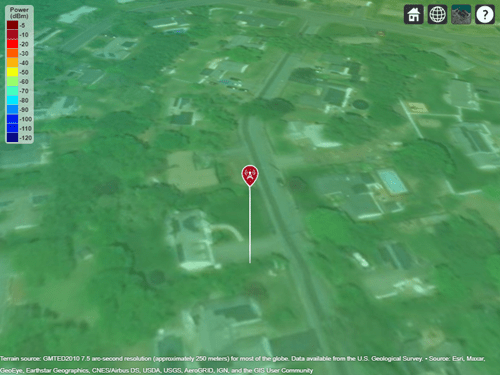### Location of New Transmitter

Use the `location` function to calculate the location of a new receiver site or transmitter site from an existing site.

Calculate a new transmitter location 1 km North of the transmitter site.

` [lat,lon] = location(tx,1000,90)`
```lat = 42.3091 ```
```lon = -71.3504 ```

Create a new transmitter site using the latitude and longitude values given by the `location `function. Use an antenna of height 30 m.

` tx2 = txsite('Name','Transmitter2','Latitude',lat,'Longitude',lon,'AntennaHeight',30)`
```tx2 = txsite with properties: Name: 'Transmitter2' Latitude: 42.3091 Longitude: -71.3504 Antenna: 'isotropic' AntennaAngle: 0 AntennaHeight: 30 SystemLoss: 0 TransmitterFrequency: 1.9000e+09 TransmitterPower: 10 ```
` show(tx2)`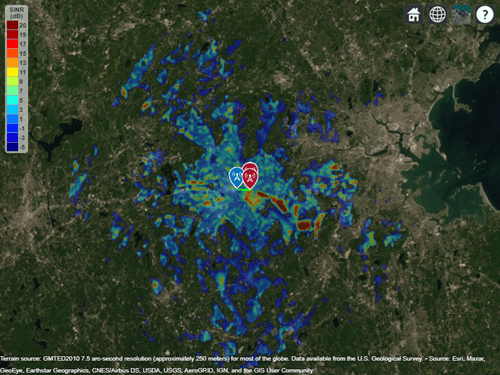### SINR of Receiver

Use the `sinr` function to calculate the SINR of the receiver. The SINR of a receiver is given by the equation:

`$\mathrm{SINR}=\frac{\mathit{S}}{\mathit{I}+\mathit{N}}$`

where,

• S = Received power of signal of interest

• I = Received power of interfering signals in the network

• N = Total received noise power

SINR is expressed in decibels.

` sinr([tx,tx2])`## Support

#### Bridging Wireless Communications Design and Testing with MATLAB

Download white paper